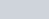If the circumference of a circle increases from 4π to 8π, then its area is
Question:

If the circumference of a circle increases from 4π to 8π, then its area is

(a) halved

(b) doubled

(c) tripled

Solution:

Let the circumference $C=4 \pi$

$\therefore 2 \pi r=4 \pi$

$\therefore r=2$

Therefore, area of the circle when radius of the circle is 2 can be calculated as below,

$\pi r^{2}=\frac{22}{7} \times 4$ ……(1)

Now when circumference is, then the radius of the circle is calculated as below,

$\therefore 2 \pi r=8 \pi$

$\therefore r=4$

Therefore, area of the circle when radius of the circle is 2 can be calculated as below,

$\pi r^{2}=\frac{22}{7} \times 16$

$\therefore \pi r^{2}=4\left(\frac{22}{7} \times 4\right)$…..(2)

Therefore, from equation (1) and (2) we can say that its area is quadrupled.

Hence, the correct answer is option $(d)$.